﻿ 基于改进遗传算法的同步发电机参数辨识

# 基于改进遗传算法的同步发电机参数辨识Parameter Identification of Synchronous Generator Based on Improved Genetic Algorithm

Abstract: A genetic algorithm for the identification of synchronous generator parameters is proposed in the case of system minor disturbance. In view of the randomness of the operation of roulette, the selection method is improved, and the problem of local optimum is avoided effectively. In order to solve the differential equation, the improved Euler method is selected, and the objective function is built according to the application of least square principle, then the improved genetic algorithm and particle swarm optimization (PSO) used for the identification of synchronous generator parameters are identified which based on PMU measured data. The identification results which compare the ad-vantages and disadvantages of two kinds of algorithm show that the improved genetic algorithm has higher identification accuracy.

1. 引言

2. 同步发电机的数学模型

1) 忽略定子磁暂态过程；

2) 计及转子d轴、q轴的暂态过程及次暂态过程。

d轴模型

$\left\{\begin{array}{l}{{T}^{\prime }}_{\text{d}0}\cdot \frac{\text{d}{{E}^{\prime }}_{\text{q}}}{\text{d}t}={E}_{f}-{{E}^{\prime }}_{\text{q}}-\frac{{x}_{\text{d}}-{{x}^{\prime }}_{\text{d}}}{{{x}^{\prime }}_{\text{d}}-{{x}^{″}}_{\text{d}}}\left({{E}^{\prime }}_{\text{q}}-{{E}^{″}}_{\text{q}}\right)\hfill \\ {{T}^{″}}_{\text{d}0}\cdot \frac{\text{d}{{E}^{″}}_{\text{q}}}{\text{d}t}={{E}^{\prime }}_{\text{q}}-{{E}^{″}}_{\text{q}}-\left({{x}^{\prime }}_{\text{d}}-{{x}^{″}}_{\text{d}}\right){i}_{\text{d}}+{{T}^{″}}_{\text{d}0}\cdot \frac{\text{d}{{E}^{\prime }}_{\text{q}}}{\text{d}t}\hfill \\ \begin{array}{l}{u}_{\text{q}}={{E}^{″}}_{\text{q}}-{{x}^{″}}_{\text{d}}{i}_{\text{d}}\\ {E}_{f}=k\cdot {u}_{f\text{d}}\end{array}\hfill \end{array}$ (1)

q轴模型

$\left\{\begin{array}{l}{{T}^{\prime }}_{\text{q}0}\cdot \frac{\text{d}{{E}^{\prime }}_{\text{d}}}{\text{d}t}=-{{E}^{\prime }}_{\text{d}}-\frac{{x}_{\text{q}}-{{x}^{\prime }}_{\text{q}}}{{{x}^{\prime }}_{\text{q}}-{{x}^{″}}_{\text{q}}}\left({{E}^{\prime }}_{\text{d}}-{{E}^{″}}_{\text{d}}\right)\hfill \\ {{T}^{″}}_{\text{q}0}\cdot \frac{\text{d}{{E}^{″}}_{\text{d}}}{\text{d}t}={{E}^{\prime }}_{\text{d}}-{{E}^{″}}_{\text{d}}+\left({{x}^{\prime }}_{\text{q}}-{{x}^{″}}_{\text{q}}\right){i}_{\text{q}}+{{T}^{″}}_{\text{q}0}\cdot \frac{\text{d}{{E}^{\prime }}_{d}}{\text{d}t}\hfill \\ {u}_{\text{d}}={{E}^{″}}_{\text{d}}+{{x}^{″}}_{\text{q}}{i}_{\text{q}}\hfill \end{array}$ (2)

3. 发电机参数辨识

${x}_{\text{q}}={U}_{\text{d}}/{I}_{\text{q}}$ (3)

${x}_{\text{d}}={x}_{\text{q}}$ (4)

3.1. 目标函数

${J}_{\text{d}}=\underset{t=1}{\overset{n}{\sum }}{\left({i}_{\text{dc}}-{i}_{\text{dm}}\right)}^{2}$ (5)

${J}_{\text{q}}=\underset{t=1}{\overset{n}{\sum }}{\left({i}_{\text{qc}}-{i}_{\text{qm}}\right)}^{2}$ (6)

3.2. 微分方程的求解

${\stackrel{¯}{y}}_{n+1}={y}_{n}+hf\left({x}_{n},{y}_{n}\right)$ (7)

${y}_{n+1}={y}_{n}+\frac{h}{2}\left[f\left({x}_{n},{y}_{n}\right)+f\left({x}_{n+1},{\stackrel{¯}{y}}_{n+1}\right)\right]$ (8)

4. 优化算法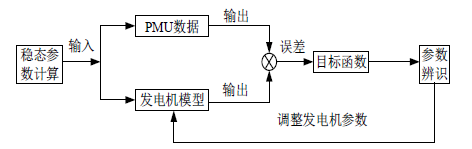Figure 1. Synchronous generator parameter identificationFigure 2. Improved Euler method

5. 算例分析验证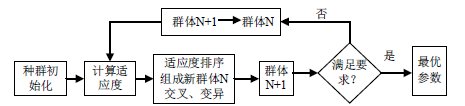Figure 3. AGA flow-process diagram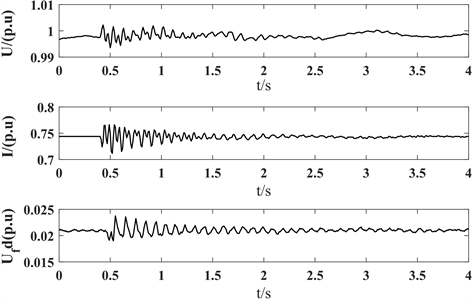Figure 4. Waveform of PMU measured dataFigure 5. Current fitting curve of direct axis-PSO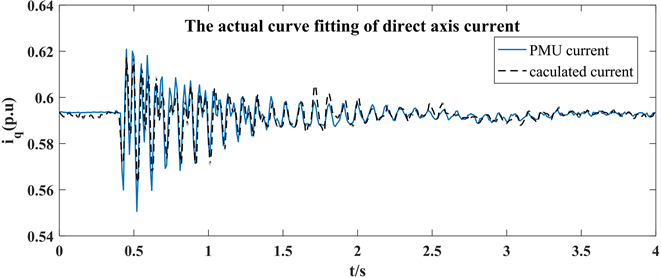Figure 6. The current fitting curve of direct axis-AGA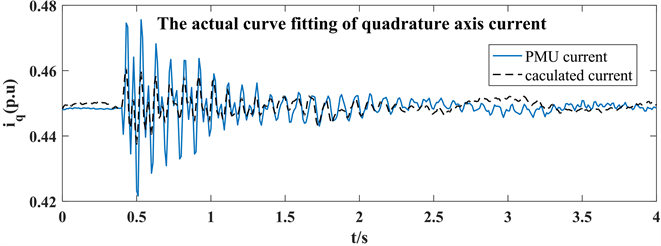Figure 7. Current fitting curve of quadrature axis-PSO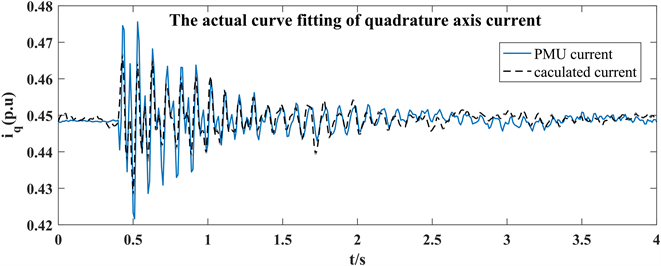Figure 8. Current fitting curve of quadrature axis-AGATable 1. Comparison of identification result-direct axisTable 2. Comparison of identification results-quadrature axis

6. 结论

 倪以信, 陈寿涛, 张宝霖. 动态电力系统的理论和分析[M]. 北京: 清华大学出版社, 2002.

 张逸, 林焱, 吴丹岳. 电能质量监测系统研究现状及发展趋势[J]. 电力系统保护与控制, 2015, 43(2): 138-147.

 陈剑, 王茂海, 刘连光. 同步发电机辨识参数对数据误差的敏感性分析[J]. 电力系统保护与控制, 2017, 45(8): 1-6.

 张子泳, 仉梦林, 李莎. 基于多目标粒子群算法的电力系统环境经济调度研究[J]. 电力系统保护与控制, 2017, 45(10): 1-10.

 程云峰, 张欣然, 陆超. 广域测量技术在电力系统中的应用研究进展[J]. 电力系统保护与控制, 2014, 42(4): 145-153.

 王茂海, 赵玉江, 齐霞, 等. 电网实际运行环境中相量测量装置性能在线评价方法[J]. 电力系统保护与控制, 2015, 43(6): 86-92.

 Khodaparast, J. and Khederzadeh, M. (2017) Least Square and Kalman Based Methods for Dynamic Phasor Estimation: A Review. Power System Protection and Control, 2, 1-7.
https://doi.org/10.1186/s41601-016-0032-y

 鞠平, 倪腊琴, 韩敬东, 等. 同步发电机参数辨识的模拟进化方法[J]. 电工技术学报, 1999, 14(5): 1.

 曹明凯, 韩超先, 马平, 等. 利用励磁电压扰动后的同步发电机参数辨识[J]. 电力系统保护与控制, 2012, 40(19): 116-120.

 鞠平. 电力系统非线性辨识[M]. 南京: 河海大学出版社, 1999.

 季文伟, 王茂海, 马平, 等. 基于实测PMU数据的风电综合负荷参数辨识[J]. 电力系统保护与控制, 2015, 43(18): 42-47.

 黄其新, 孙黎霞, 甄威, 等. 鞠平同步发电机参数辨识的蚁群算法及扰动分析[J]. 电力自动化设备, 2009: 11.

 孙黎霞, 鞠平, 高运华, 等. 基于Park模型的同步发电机参数辨识[J]. 中国电机工程学报, 2009, 29(19): 50-56.

 林国汉, 章兢, 刘朝华, 等. 改进综合学习粒子群算法的PMSM 参数辨识[J]. 电机与控制学报, 2015, 19(1): 51-57.

 伍双喜, 吴文传, 张伯明, 等. 用PMU实测数据辨识同步发电机参数的关键问题[J]. 电力系统自动化, 2012, 36(17): 50-55.

 沈善德. 电力系统辨识[M]. 北京: 水利电力出版社, 1995.

 李志强, 汤涌, 罗炜. 基于时频变换的同步发电机参数辨识中权函数选择与误差分析[J]. 中国电机工程学报, 2016, 36(3): 828-835.

 汤涌. 基于电机参数的同步电机模型[J]. 电网技术, 2007, 31(12): 47-51.

 韩英铎, 严剑峰, 谢小荣, 等. 电力系统机电暂态过程主导动态参数的在线辨识[J]. 中国电机工程学报, 2006, 26(2): 1-6.

 刘鹏程, 李新利. 基于多种群遗传算法的含分布式电源的配电网故障区段定位算法[J]. 电力系统保护与控制, 2016, 44(2): 36-41.

Top